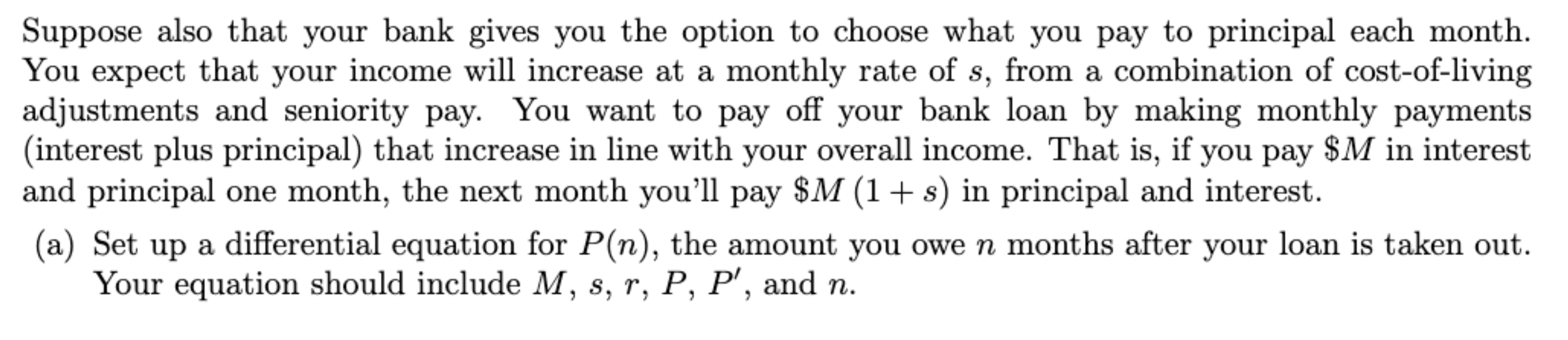# Question Suppose also that your bank gives you the option to choose what you pay to principal each month. You expect that your income will increase at a monthly rate of $$s$$, from a combination of cost-of-living adjustments and seniority pay. You want to pay off your bank loan by making monthly payments (interest plus principal) that increase in line with your overall income. That is, if you pay $$\ M$$ in interest and principal one month, the next month you'll pay $$\ M(1+s)$$ in principal and interest. (a) Set up a differential equation for $$P(n)$$, the amount you owe $$n$$ months after your loan is taken out. Your equation should include $$M, s, r, P, P^{\prime}$$, and $$n$$.Transcribed Image Text: Suppose also that your bank gives you the option to choose what you pay to principal each month. You expect that your income will increase at a monthly rate of $$s$$, from a combination of cost-of-living adjustments and seniority pay. You want to pay off your bank loan by making monthly payments (interest plus principal) that increase in line with your overall income. That is, if you pay $$\ M$$ in interest and principal one month, the next month you'll pay $$\ M(1+s)$$ in principal and interest. (a) Set up a differential equation for $$P(n)$$, the amount you owe $$n$$ months after your loan is taken out. Your equation should include $$M, s, r, P, P^{\prime}$$, and $$n$$.
Transcribed Image Text: Suppose also that your bank gives you the option to choose what you pay to principal each month. You expect that your income will increase at a monthly rate of $$s$$, from a combination of cost-of-living adjustments and seniority pay. You want to pay off your bank loan by making monthly payments (interest plus principal) that increase in line with your overall income. That is, if you pay $$\ M$$ in interest and principal one month, the next month you'll pay $$\ M(1+s)$$ in principal and interest. (a) Set up a differential equation for $$P(n)$$, the amount you owe $$n$$ months after your loan is taken out. Your equation should include $$M, s, r, P, P^{\prime}$$, and $$n$$.